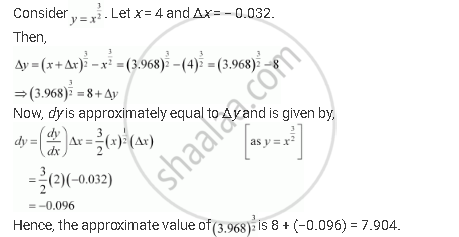Share

# Using Differentials, Find the Approximate Value of the Following up to 3 Places of Decimal (3.968)^(3/2) - Mathematics

#### Question

Using differentials, find the approximate value of the following up to 3 places of decimal

(3.968)^(3/2)

#### Solution

(3.968)^(3/2)Is there an error in this question or solution?
Using Differentials, Find the Approximate Value of the Following up to 3 Places of Decimal (3.968)^(3/2) Concept: Approximations.